# PSAT Math : How to find the surface area of a sphere

## Example Questions

### Example Question #1 : How To Find The Surface Area Of A Sphere

A spherical orange fits snugly inside a small cubical box such that each of the six walls of the box just barely touches the surface of the orange.  If the volume of the box is 64 cubic inches, what is the surface area of the orange in square inches?

32π

256π

128π

64π

16π

16π

Explanation:

The volume of a cube is found by V = s3.  Since V = 64, s = 4.  The side of the cube is the same as the diameter of the sphere.  Since d = 4, r = 2.  The surface area of a sphere is found by SA = 4π(r2) = 4π(22) = 16π.

### Example Question #1 : How To Find The Surface Area Of A Sphere

A solid sphere is cut in half to form two solid hemispheres. What is the ratio of the surface area of one of the hemispheres to the surface area of the entire sphere before it was cut?

1

1/2

2/3

3/4

3/2

3/4

Explanation:

The surface area of the sphere before it was cut is equal to the following:

surface area of solid sphere = 4πr2, where r is the length of the radius.

Each hemisphere will have the following shape:In order to determine the surface area of the hemisphere, we must find the surface area of the flat region and the curved region. The flat region will have a surface area equal to the area of a circle with radius r.

area of flat part of hemisphere = πr2

The surface area of the curved portion of the hemisphere will equal one-half of the surface area of the uncut sphere, which we established to be 4πr2.

area of curved part of hemisphere = (1/2)4πr= 2πr2

The total surface area of the hemisphere will be equal to the sum of the surface areas of the flat part and curved part of the hemisphere.

total surface area of hemisphere = πr+ 2πr= 3πr2

Finally, we must find the ratio of the surface area of the hemisphere to the surface area of the uncut sphere.

ratio = (3πr2)/(4πr2) = 3/4

### Example Question #1 : Spheres

The volume of a sphere is 2304π in3.  What is the surface area of this sphere in square inches?

576π

216π

144π

36π

576π

Explanation:

To solve this, we must first begin by finding the radius of the sphere. To do this, recall that the volume of a sphere is:

V = (4/3)πr3

For our data, we can say:

2304π = (4/3)πr3; 2304 = (4/3)r3; 6912 = 4r3; 1728 = r3; 12 * 12 * 12 = r3; r = 12

Now, based on this, we can ascertain the surface area using the equation:

A = 4πr2

For our data, this is:

A = 4π*122 = 576π

### Example Question #11 : How To Find Surface Area

A sphere has its center at the origin.  A point on its surface is found on the x-y axis at (6,8).  In square units, what is the surface area of this sphere?

200π

400π

40π

(400/3)π

400π

Explanation:

To find the surface area, we must first find the radius.  Based on our description, this passes from (0,0) to (6,8).  This can be found using the distance formula:

62 + 82 = r2; r2 = 36 + 64; r2 = 100; r = 10

It should be noted that you could have quickly figured this out by seeing that (6,8) is the hypotenuse of a 6-8-10 triangle (which is a multiple of the "easy" 3-4-5).

The rest is easy.  The surface area of the sphere is defined by:

A = 4πr2 = 4 * 100 * π = 400π

### Example Question #91 : Solid Geometry

A sphere is perfectly contained within a cube that has a surface area of 726 square units. In square units, what is the surface area of the sphere?

11π

30.25π

121π

484π

121π

Explanation:

To begin, we must determine the dimensions of the cube. To do this, recall that the surface area of a cube is made up of six squares and is thus defined as: A = 6s2, where s is one of the sides of the cube. For our data, this gives us:

726 = 6s2; 121 = s2; s = 11

Now, if the sphere is contained within the cube, that means that 11 represents the diameter of the sphere. Therefore, the radius of the sphere is 5.5 units. The surface area of a sphere is defined as: A = 4πr2. For our data, that would be:

A = 4π * 5.52 = 30.25 * 4 * π = 121π

### Example Question #1 : How To Find The Surface Area Of A Sphere

The area of a circle with radius 4 divided by the surface area of a sphere with radius 2 is equal to:

π

2

3

0.5

1

1

Explanation:

The surface area of a sphere is 4πr2. The area of a circle is πr2. 16/16 is equal to 1.

### Example Question #1 : How To Find The Surface Area Of A Sphere

What is the ratio of the surface area of a cube to the surface area of a sphere inscribed within it?

π/3

3/π

6/π

4/π

2π

6/π

Explanation:

Let's call the radius of the sphere r. The formula for the surface area of a sphere (A) is given below:

A = 4πr2

Because the sphere is inscribed inside the cube, the diameter of the sphere is equal to the side length of the cube. Because the diameter is twice the length of the radius, the diameter of the sphere is 2r. This means that the side length of the cube is also 2r

The surface area for a cube is given by the following formula, where s represents the length of each side of the cube:

surface area of cube = 6s2

The formula for surface area of a cube comes from the fact that each face of the cube has an area of s2, and there are 6 faces total on a cube.

Since we already determined that the side length of the cube is the same as 2r, we can replace s with 2r.

surface area of cube = 6(2r)= 6(2r)(2r) = 24r2.

We are asked to find the ratio of the surface area of the cube to the surface area of the sphere. This means we must divide the surface area of the cube by the surface area of the sphere.

ratio = (24r2)/(4πr2)

The rterm cancels in the numerator and denominator. Also, 24/4 simplifes to 6.

ratio = (24r2)/(4πr2) = 6/π

### Example Question #1 : How To Find The Surface Area Of A Sphere

What is the surface area of a hemisphere with a diameter of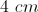?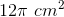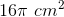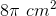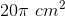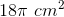Explanation:

A hemisphere is half of a sphere.  The surface area is broken into two parts:  the spherical part and the circular base.

The surface area of a sphere is given by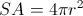.

So the surface area of the spherical part of a hemisphere is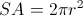The area of the circular base is given by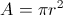.  The radius to use is half the diameter, or 2 cm.

### All PSAT Math Resources